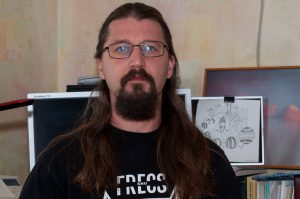Phd, assistant, 1979.

In 2001 г. he graduated from the Faculty of Mechanics and Mathematics of Kyiv National Taras Shevchenko University.

Since 2002 he has been working as an assistant at the Department of Mathematics and Theoretical Radiophysics. He gives lectures at the course “Fundamentals of Programming” for students of the Institute of Geology, conducts seminars on mathematical analysis, analytical geometry and linear algebra, differential equations, probability theory, laboratory classes in numerical methods, the basics of programming and software engineering, and also works in the preparatory department.

Main scientific interests: statistics of stochastic processes.

Author of 11 articles.